# CMSC 56 | Lecture 6: Sets & Set Operations

25. Apr 2019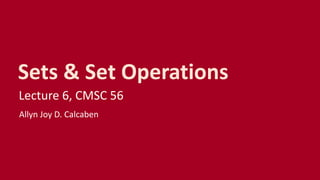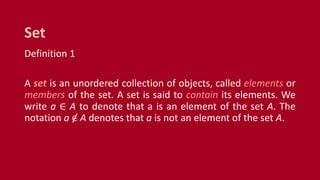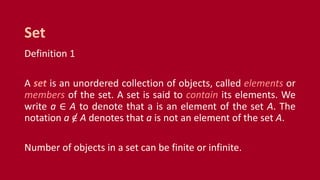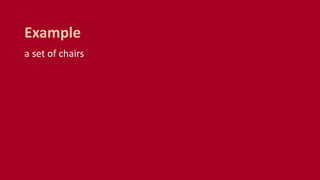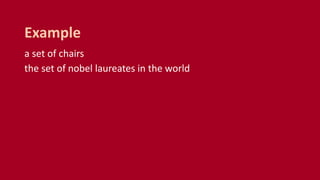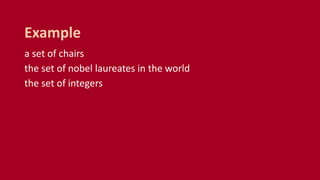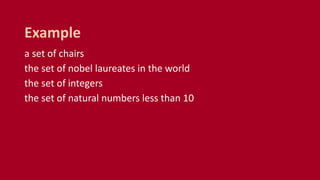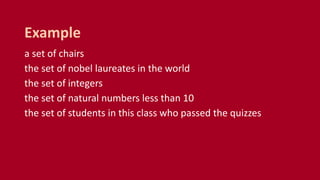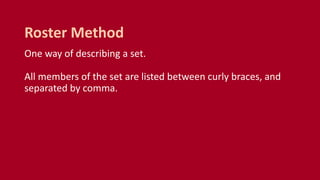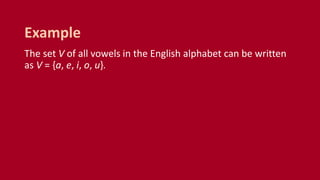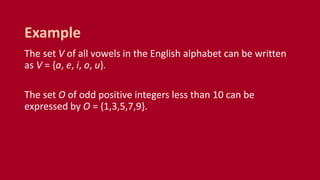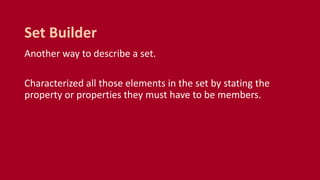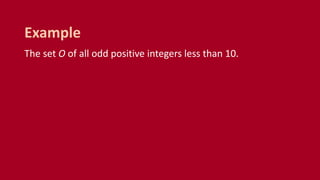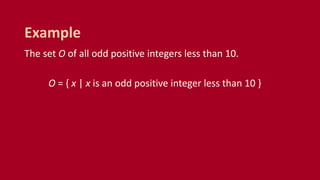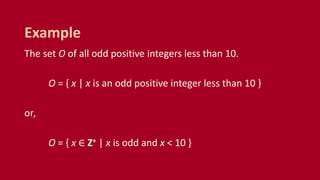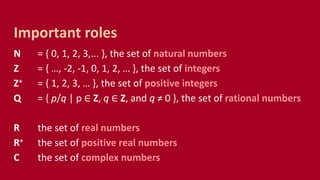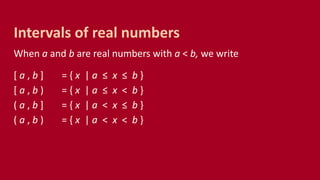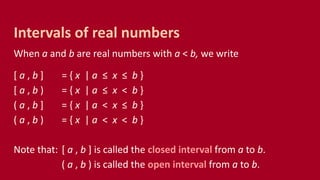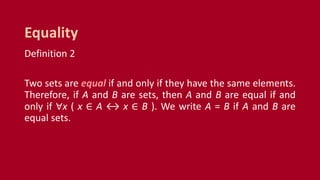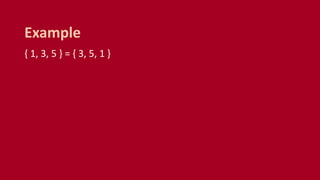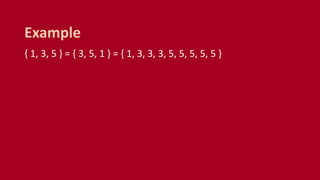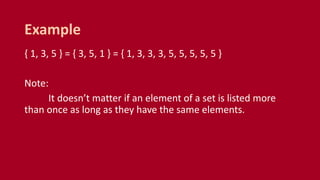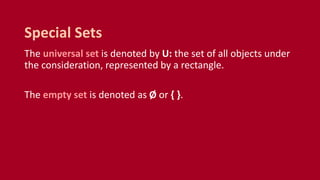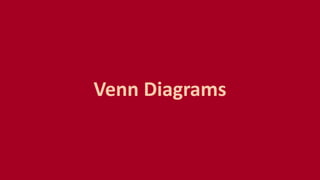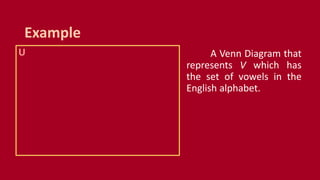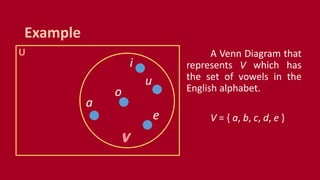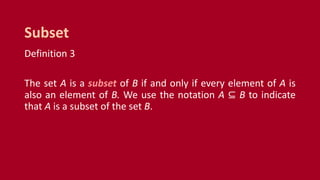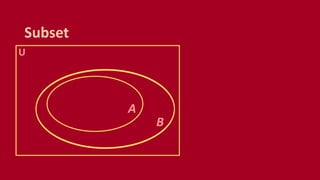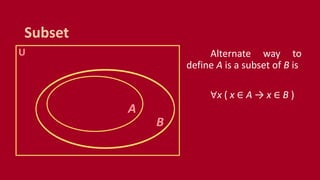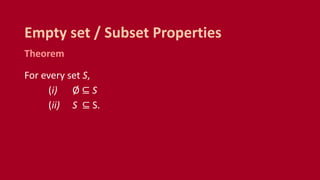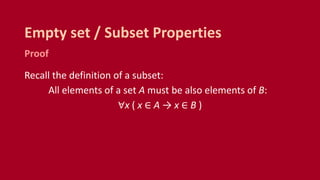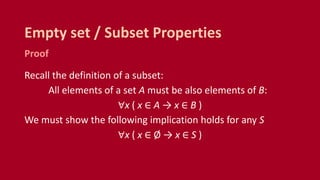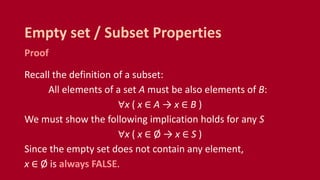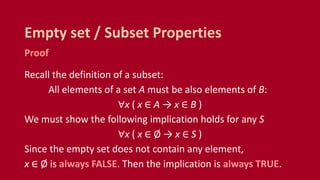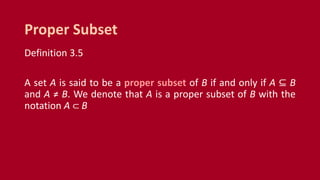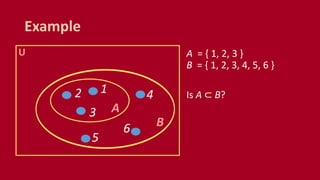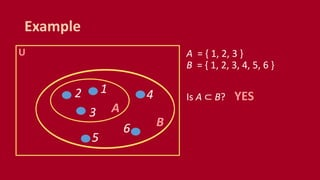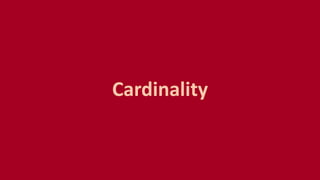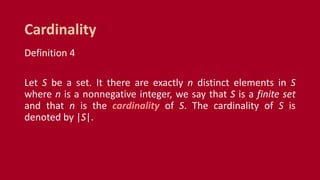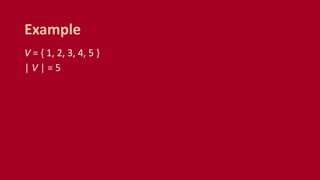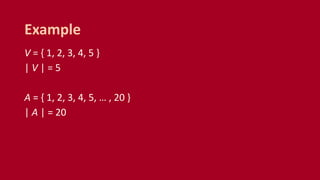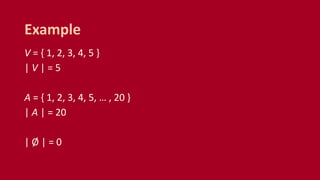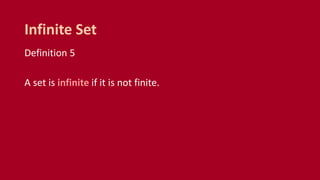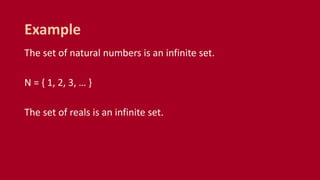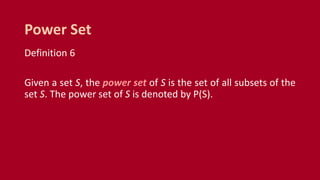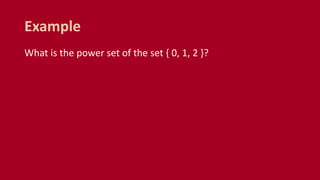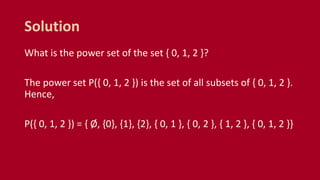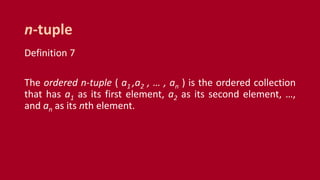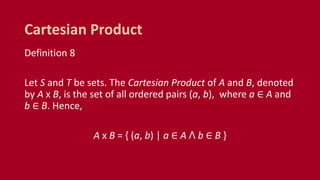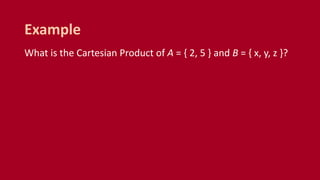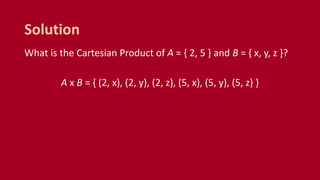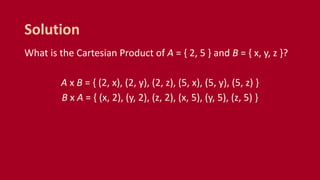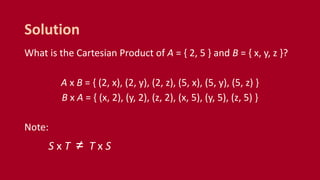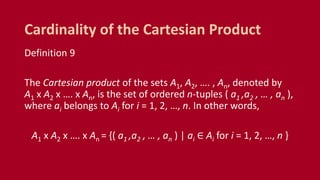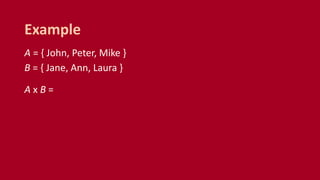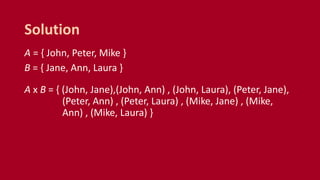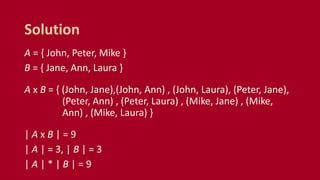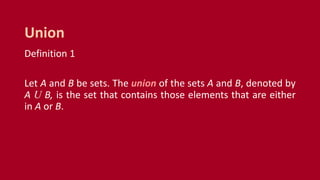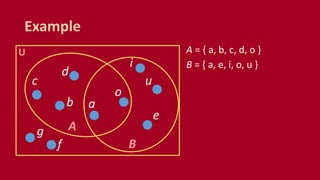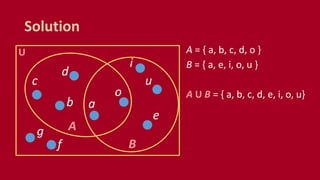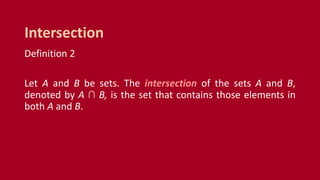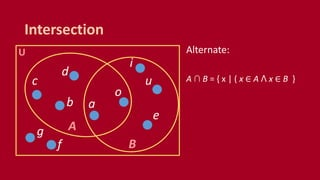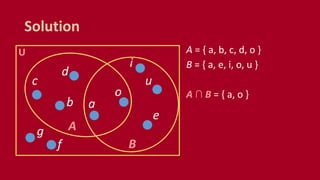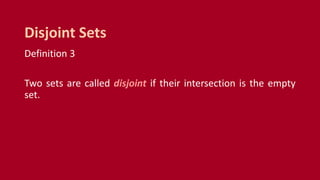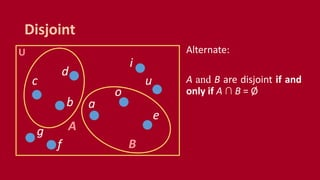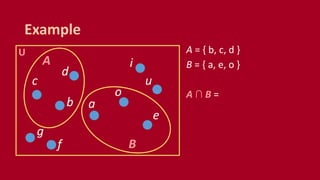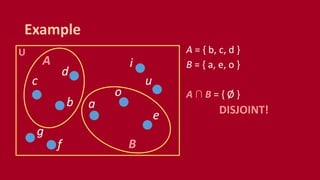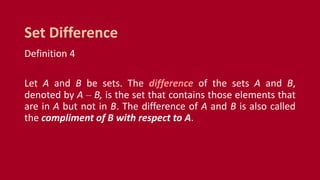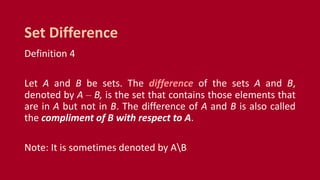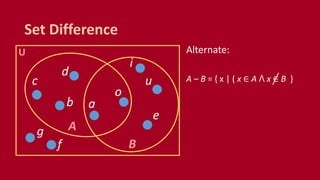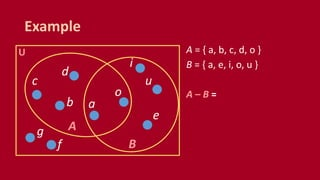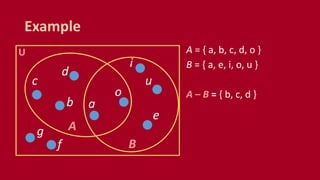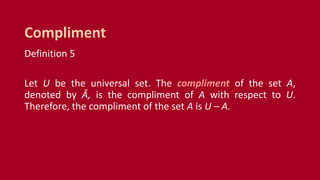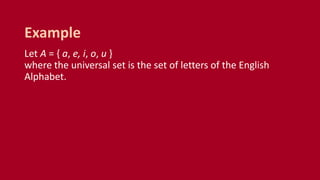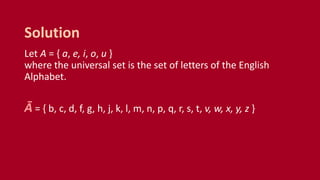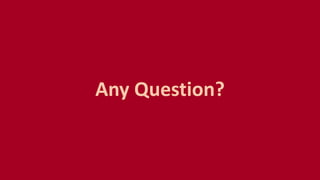1 von 80

### CMSC 56 | Lecture 6: Sets & Set Operations

• 1. Sets & Set Operations Lecture 6, CMSC 56 Allyn Joy D. Calcaben
• 2. Definition 1 A set is an unordered collection of objects, called elements or members of the set. A set is said to contain its elements. We write a ∈ A to denote that a is an element of the set A. The notation a ∈ A denotes that a is not an element of the set A. Set
• 3. Definition 1 A set is an unordered collection of objects, called elements or members of the set. A set is said to contain its elements. We write a ∈ A to denote that a is an element of the set A. The notation a ∈ A denotes that a is not an element of the set A. Number of objects in a set can be finite or infinite. Set
• 4. a set of chairs Example
• 5. a set of chairs the set of nobel laureates in the world Example
• 6. a set of chairs the set of nobel laureates in the world the set of integers Example
• 7. a set of chairs the set of nobel laureates in the world the set of integers the set of natural numbers less than 10 Example
• 8. a set of chairs the set of nobel laureates in the world the set of integers the set of natural numbers less than 10 the set of students in this class who passed the quizzes Example
• 9. One way of describing a set. All members of the set are listed between curly braces, and separated by comma. Roster Method
• 10. The set V of all vowels in the English alphabet can be written as V = {a, e, i, o, u}. Example
• 11. The set V of all vowels in the English alphabet can be written as V = {a, e, i, o, u}. The set O of odd positive integers less than 10 can be expressed by O = {1,3,5,7,9}. Example
• 12. Another way to describe a set. Characterized all those elements in the set by stating the property or properties they must have to be members. Set Builder
• 13. The set O of all odd positive integers less than 10. Example
• 14. The set O of all odd positive integers less than 10. O = { x | x is an odd positive integer less than 10 } Example
• 15. The set O of all odd positive integers less than 10. O = { x | x is an odd positive integer less than 10 } or, O = { x ∈ Z+ | x is odd and x < 10 } Example
• 16. N = { 0, 1, 2, 3,... }, the set of natural numbers Z = { …, -2, -1, 0, 1, 2, … }, the set of integers Z+ = { 1, 2, 3, … }, the set of positive integers Q = { p/q | p ∈ Z, q ∈ Z, and q ≠ 0 }, the set of rational numbers R the set of real numbers R+ the set of positive real numbers C the set of complex numbers Important roles
• 17. When a and b are real numbers with a < b, we write [ a , b ] = { x | a ≤ x ≤ b } [ a , b ) = { x | a ≤ x < b } ( a , b ] = { x | a < x ≤ b } ( a , b ) = { x | a < x < b } Intervals of real numbers
• 18. When a and b are real numbers with a < b, we write [ a , b ] = { x | a ≤ x ≤ b } [ a , b ) = { x | a ≤ x < b } ( a , b ] = { x | a < x ≤ b } ( a , b ) = { x | a < x < b } Note that: [ a , b ] is called the closed interval from a to b. ( a , b ) is called the open interval from a to b. Intervals of real numbers
• 19. Definition 2 Two sets are equal if and only if they have the same elements. Therefore, if A and B are sets, then A and B are equal if and only if ∀x ( x ∈ A ↔ x ∈ B ). We write A = B if A and B are equal sets. Equality
• 20. { 1, 3, 5 } = { 3, 5, 1 } Example
• 21. { 1, 3, 5 } = { 3, 5, 1 } = { 1, 3, 3, 3, 5, 5, 5, 5, 5 } Example
• 22. { 1, 3, 5 } = { 3, 5, 1 } = { 1, 3, 3, 3, 5, 5, 5, 5, 5 } Note: It doesn’t matter if an element of a set is listed more than once as long as they have the same elements. Example
• 23. The universal set is denoted by U: the set of all objects under the consideration, represented by a rectangle. The empty set is denoted as Ø or { }. Special Sets
• 25. U Example A Venn Diagram that represents V which has the set of vowels in the English alphabet.
• 26. U Example A Venn Diagram that represents V which has the set of vowels in the English alphabet. V = { a, b, c, d, e } a e i o u VV
• 27. Definition 3 The set A is a subset of B if and only if every element of A is also an element of B. We use the notation A ⊆ B to indicate that A is a subset of the set B. Subset
• 29. Subset U B A Alternate way to define A is a subset of B is ∀x ( x ∈ A → x ∈ B )
• 30. Theorem For every set S, (i) Ø ⊆ S (ii) S ⊆ S. Empty set / Subset Properties
• 31. Proof Recall the definition of a subset: All elements of a set A must be also elements of B: ∀x ( x ∈ A → x ∈ B ) Empty set / Subset Properties
• 32. Proof Recall the definition of a subset: All elements of a set A must be also elements of B: ∀x ( x ∈ A → x ∈ B ) We must show the following implication holds for any S ∀x ( x ∈ Ø → x ∈ S ) Empty set / Subset Properties
• 33. Proof Recall the definition of a subset: All elements of a set A must be also elements of B: ∀x ( x ∈ A → x ∈ B ) We must show the following implication holds for any S ∀x ( x ∈ Ø → x ∈ S ) Since the empty set does not contain any element, x ∈ Ø is always FALSE. Empty set / Subset Properties
• 34. Proof Recall the definition of a subset: All elements of a set A must be also elements of B: ∀x ( x ∈ A → x ∈ B ) We must show the following implication holds for any S ∀x ( x ∈ Ø → x ∈ S ) Since the empty set does not contain any element, x ∈ Ø is always FALSE. Then the implication is always TRUE. Empty set / Subset Properties
• 35. Definition 3.5 A set A is said to be a proper subset of B if and only if A ⊆ B and A ≠ B. We denote that A is a proper subset of B with the notation A ⊂ B Proper Subset
• 36. Example U B A A = { 1, 2, 3 } B = { 1, 2, 3, 4, 5, 6 } Is A ⊂ B?12 3 4 5 6
• 37. Example U B A A = { 1, 2, 3 } B = { 1, 2, 3, 4, 5, 6 } Is A ⊂ B? YES 12 3 4 5 6
• 39. Definition 4 Let S be a set. It there are exactly n distinct elements in S where n is a nonnegative integer, we say that S is a finite set and that n is the cardinality of S. The cardinality of S is denoted by |S|. Cardinality
• 40. V = { 1, 2, 3, 4, 5 } | V | = 5 Example
• 41. V = { 1, 2, 3, 4, 5 } | V | = 5 A = { 1, 2, 3, 4, 5, … , 20 } | A | = 20 Example
• 42. V = { 1, 2, 3, 4, 5 } | V | = 5 A = { 1, 2, 3, 4, 5, … , 20 } | A | = 20 | Ø | = 0 Example
• 43. Definition 5 A set is infinite if it is not finite. Infinite Set
• 44. The set of natural numbers is an infinite set. N = { 1, 2, 3, … } The set of reals is an infinite set. Example
• 46. Definition 6 Given a set S, the power set of S is the set of all subsets of the set S. The power set of S is denoted by P(S). Power Set
• 47. What is the power set of the set { 0, 1, 2 }? Example
• 48. What is the power set of the set { 0, 1, 2 }? The power set P({ 0, 1, 2 }) is the set of all subsets of { 0, 1, 2 }. Hence, P({ 0, 1, 2 }) = { Ø, {0}, {1}, {2}, { 0, 1 }, { 0, 2 }, { 1, 2 }, { 0, 1, 2 }} Solution
• 50. Definition 7 The ordered n-tuple ( a1 ,a2 , … , an ) is the ordered collection that has a1 as its first element, a2 as its second element, …, and an as its nth element. n-tuple
• 51. Definition 8 Let S and T be sets. The Cartesian Product of A and B, denoted by A x B, is the set of all ordered pairs (a, b), where a ∈ A and b ∈ B. Hence, A x B = { (a, b) | a ∈ A ꓥ b ∈ B } Cartesian Product
• 52. What is the Cartesian Product of A = { 2, 5 } and B = { x, y, z }? Example
• 53. What is the Cartesian Product of A = { 2, 5 } and B = { x, y, z }? A x B = { (2, x), (2, y), (2, z), (5, x), (5, y), (5, z) } Solution
• 54. What is the Cartesian Product of A = { 2, 5 } and B = { x, y, z }? A x B = { (2, x), (2, y), (2, z), (5, x), (5, y), (5, z) } B x A = { (x, 2), (y, 2), (z, 2), (x, 5), (y, 5), (z, 5) } Solution
• 55. What is the Cartesian Product of A = { 2, 5 } and B = { x, y, z }? A x B = { (2, x), (2, y), (2, z), (5, x), (5, y), (5, z) } B x A = { (x, 2), (y, 2), (z, 2), (x, 5), (y, 5), (z, 5) } Note: S x T ≠ T x S Solution
• 56. Definition 9 The Cartesian product of the sets A1, A2, …. , An, denoted by A1 x A2 x …. x An, is the set of ordered n-tuples ( a1 ,a2 , … , an ), where ai belongs to Ai for i = 1, 2, …, n. In other words, A1 x A2 x …. x An = {( a1 ,a2 , … , an ) | ai ∈ Ai for i = 1, 2, …, n } Cardinality of the Cartesian Product
• 57. A = { John, Peter, Mike } B = { Jane, Ann, Laura } A x B = Example
• 58. A = { John, Peter, Mike } B = { Jane, Ann, Laura } A x B = { (John, Jane),(John, Ann) , (John, Laura), (Peter, Jane), (Peter, Ann) , (Peter, Laura) , (Mike, Jane) , (Mike, Ann) , (Mike, Laura) } Solution
• 59. A = { John, Peter, Mike } B = { Jane, Ann, Laura } A x B = { (John, Jane),(John, Ann) , (John, Laura), (Peter, Jane), (Peter, Ann) , (Peter, Laura) , (Mike, Jane) , (Mike, Ann) , (Mike, Laura) } | A x B | = 9 | A | = 3, | B | = 3 | A | * | B | = 9 Solution
• 61. Definition 1 Let A and B be sets. The union of the sets A and B, denoted by A U B, is the set that contains those elements that are either in A or B. Union
• 62. U Example A = { a, b, c, d, o } B = { a, e, i, o, u } a e i o u A B b c d f g
• 63. U Solution A = { a, b, c, d, o } B = { a, e, i, o, u } A U B = { a, b, c, d, e, i, o, u} a e i o u A B b c d f g
• 64. Definition 2 Let A and B be sets. The intersection of the sets A and B, denoted by A ∩ B, is the set that contains those elements in both A and B. Intersection
• 65. U Intersection Alternate: A ∩ B = { x | ( x ∈ A ꓥ x ∈ B } a e i o u A B b c d f g
• 66. U Example A = { a, b, c, d, o } B = { a, e, i, o, u } a e i o u A B b c d f g
• 67. U Solution A = { a, b, c, d, o } B = { a, e, i, o, u } A ∩ B = { a, o } a e i o u A B b c d f g
• 68. Definition 3 Two sets are called disjoint if their intersection is the empty set. Disjoint Sets
• 69. U Disjoint Alternate: A and B are disjoint if and only if A ∩ B = Ø a e i o u A B b c d f g
• 70. U Example A = { b, c, d } B = { a, e, o } A ∩ B = a e i o u A B b c d f g
• 71. U Example A = { b, c, d } B = { a, e, o } A ∩ B = { Ø } DISJOINT!a e i o u A B b c d f g
• 72. Definition 4 Let A and B be sets. The difference of the sets A and B, denoted by A – B, is the set that contains those elements that are in A but not in B. The difference of A and B is also called the compliment of B with respect to A. Set Difference
• 73. Definition 4 Let A and B be sets. The difference of the sets A and B, denoted by A – B, is the set that contains those elements that are in A but not in B. The difference of A and B is also called the compliment of B with respect to A. Note: It is sometimes denoted by AB Set Difference
• 74. U Set Difference Alternate: A – B = { x | ( x ∈ A ꓥ x ∈ B } a e i o u A B b c d f g
• 75. U Example A = { a, b, c, d, o } B = { a, e, i, o, u } A – B = a e i o u A B b c d f g
• 76. U Example A = { a, b, c, d, o } B = { a, e, i, o, u } A – B = { b, c, d } a e i o u A B b c d f g
• 77. Definition 5 Let U be the universal set. The compliment of the set A, denoted by Ā, is the compliment of A with respect to U. Therefore, the compliment of the set A is U – A. Compliment
• 78. Let A = { a, e, i, o, u } where the universal set is the set of letters of the English Alphabet. Example
• 79. Let A = { a, e, i, o, u } where the universal set is the set of letters of the English Alphabet. Ā = { b, c, d, f, g, h, j, k, l, m, n, p, q, r, s, t, v, w, x, y, z } Solution

### Hinweis der Redaktion

1. We see that A ⊆ B if and only if the quantification ∀x ( x ∈ A → x ∈ B ) is true. Note that to show that A is not a subset of B we need only ﬁnd one element x ∈ A with x ∈ B. Such an x is a counterexample to the claim that x ∈ A implies x ∈ B.
2. We see that A ⊆ B if and only if the quantification ∀x ( x ∈ A → x ∈ B ) is true. Note that to show that A is not a subset of B we need only ﬁnd one element x ∈ A with x ∈ B. Such an x is a counterexample to the claim that x ∈ A implies x ∈ B.
3. We see that A ⊆ B if and only if the quantification ∀x ( x ∈ A → x ∈ B ) is true. Note that to show that A is not a subset of B we need only ﬁnd one element x ∈ A with x ∈ B. Such an x is a counterexample to the claim that x ∈ A implies x ∈ B.
4. We see that A ⊆ B if and only if the quantification ∀x ( x ∈ A → x ∈ B ) is true. Note that to show that A is not a subset of B we need only ﬁnd one element x ∈ A with x ∈ B. Such an x is a counterexample to the claim that x ∈ A implies x ∈ B.
5. Note that the empty set and the set itself are members of this set of subsets.
6. Sets are used to represent unordered collections. Ordered-n tuples are used to represent an ordered collection.